# Becker-Gottlieb transfer

(diff) ← Older revision | Latest revision (diff) | Newer revision → (diff)

An-mapping from the base to the total space of a fibration. It thus induces homomorphisms on homology and cohomology, which are also called Becker–Gottlieb transfers.

Suppose thatis a Hurewicz fibration (cf. Covering homotopy) whose fibreis homotopy equivalent to a compact CW-complex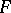. The Becker–Gottlieb transfer is an-mapping, which means that there is a mapping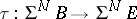defined between the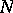th suspensions ofandfor some(cf. Suspension). Thus,induces a homomorphism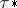on any homology theory and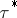on any cohomology theory.

This gives striking relations on homology and cohomology, respectively:(a1)

where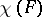denotes multiplication by the Euler–Poincaré number of.

The Becker–Gottlieb transfer was discovered in the mid-1970s. It generalizes the transfer for finite covering spaces (is a finite set of points), which had been well-known since the 1940s and which was a generalization of a group theory transfer from the 1920s.

Since the discovery of the Becker–Gottlieb transfer, other transfers have been discovered which satisfy equations (a1) with the Euler–Poincaré number replaced by another elementary topological invariant. Thus,(a2)

wheredenotes multiplication by an integer, which can be a Lefschetz number, a coincidence number, a fixed-point index, a vector field index, or an intersection number. For any mapping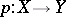, the greatest common divisor of all the integersassociated to a transfer is the Brouwer degree ofwhenandare manifolds of the same dimension (cf. also Degree of a mapping). Some of these transfers are induced by-mappings, and others are not.

Several mathematicians contributed to the discovery of these other transfers, most of which either generalize some or all of the Becker–Gottlieb transfer. Dold's fixed-point transfer was discovered independently of the Becker–Gottlieb transfer, which it generalizes. It is sometimes called the Becker–Gottlieb–Dold transfer.

How to Cite This Entry:
Becker-Gottlieb transfer. Encyclopedia of Mathematics. URL: http://encyclopediaofmath.org/index.php?title=Becker-Gottlieb_transfer&oldid=11358
This article was adapted from an original article by D.H. Gottlieb (originator), which appeared in Encyclopedia of Mathematics - ISBN 1402006098. See original article